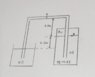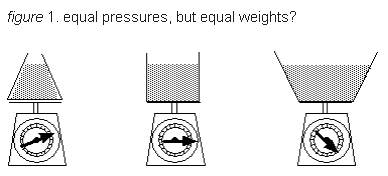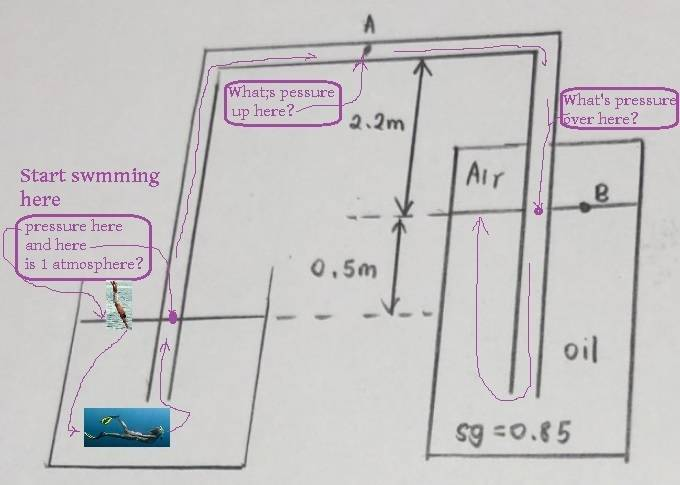• Brownie

## Homework Statement

The points of interest are at point A and B, I want to calculate the pressures at that point. I am working in gage pressure so we can ignore atmospheric pressure.

## Homework Equations

P = pgh
P = sg x specific weight of water x height

## The Attempt at a Solution

Attempting a first because I am stuck at a.
I used Pascal paradox. The level I am using is at the level of the open tank oil level. Left side is p1 and right is p2.

Pressure at point 1 is = 0 (as I’m working in gage pressure)

I’m stuck at point 2.

I think I would need a to solve b

#### Attachments

•CA4CE9C7-983A-4FC2-92E7-E82B8E945913.jpeg
22.7 KB · Views: 350

## Homework Statement

The points of interest are at point A and B, I want to calculate the pressures at that point. I am working in gage pressure so we can ignore atmospheric pressure.

## Homework Equations

P = pgh
P = sg x specific weight of water x height

## The Attempt at a Solution

Attempting a first because I am stuck at a.
I used Pascal paradox. The level I am using is at the level of the open tank oil level. Left side is p1 and right is p2.

Pressure at point 1 is = 0 (as I’m working in gage pressure)

I’m stuck at point 2.

I think I would need a to solve b
You have left out a lot here, so I am going to make some assumptions:
1) The system is in equilibrium
2) The pipe is full of oil
3) The surface area in the two tanks is the same

Please let me know if those assumptions are not correct. I am not sure how you plan to use the hydrostatic paradox here because the levels in the two tanks are not equal. Let's talk about the pressure at point B. If we assume that the two tanks have the same area, then you can equate the weight of the oil in the tank on the left plus the weight of the entire atmosphere above it with the weight of the oil and air in the tank on the right. You should be able to solve for the pressure at point B.

I agree with @tnich --- if this is Pascal's Paradox i don't see how it relates to your question...................................

The mental trick i'd use is to imagine myself very small, swimming through your apparatus and carrying an absolute pressure gage.old jim